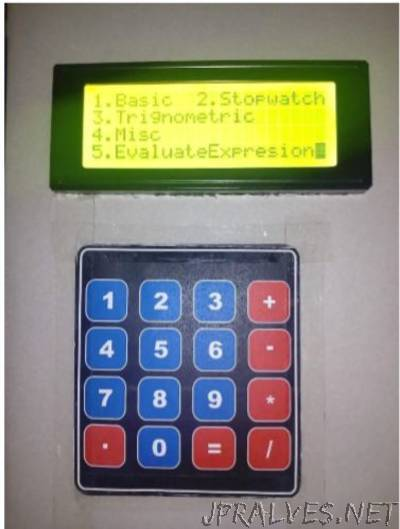This project is aimed at making an LCD based Digital Calculator performing various functions. A Calculator is an electronic device which will evaluate various mathematical expressions and calculations. The calculator of this particular project was designed using Tiva TM4c1233GXL micro-controller. The calculator first gives the user various operations to be performed. The user selects the particular operation to be performed, enters the desired arguments and the calculator evaluates the operation and displays the result on the LCD Screen. This particular calculator can perform the following operations: Arithmetic Functions; Trigonometric Functions; Conversion of numbers between different bases; Evaluation of Infix Expressions; Factorial of a number; Calculation of the nth power of a number.”